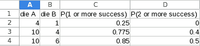# Need a Probability Equation for Dice Outcomes

##### New member
Hello,

I'm looking for assistance in devising an equation to determine the probability of outcomes on dice pools. I'm having two issues in doing it myself, because I'm not looking for the probability of the sums of the dice, but the likelihood that each group of dice will have dice that roll equal to or greater than a given threshold (4 or 8). These thresholds represent "success". Greater than 4 is 1 success. Greater than 8 is 2 successes. The dice we use are d4, d6, d8, and/or d10.

The system scales the dice. We start with 1d4, move up to 1d6, then 1d8, and 1d10. After this, we add a second die that scales. So: 1d10 & 1d4, 1d10 & 1d6, etc. The same thing happens after 2d10, all the way up to 5d10.

So, for example, in 1d10&1d4, the chance of one of them rolling => 4 (1 success) is 77.50% (70% for the 1d10 to land 4-10, 7.5% chance for the 1d4 to land 4 and the 1d10 to land 1-3) if I've done my math right. The chance of 2 successes (1d10 >= 8 OR 1d10 = {4..7} & 1d10 = 4) is 30%. I think.

It's been slow going, but I'm hoping to speed up the process.

#### Cubist

##### Full Member
...The chance of 2 successes (1d10 >= 8 OR 1d10 = {4..7} & 1d10 = 4) is 30%. I think.
You made a mistake above. (30% for 1d10 >= 8 OR 10% for 1d10 = {4..7} & 1d4 = 4) which comes to 40%

...but I'm hoping to speed up the process.
You could make a spreadsheet like this...I did this by using the spreadsheet's min/max functions. Note that having die B as one-sided is equivalent to having just one die A. I'm going to give you quite a lot of help since I suspect that this isn't a math question from your school/ college. (Are you designing a game of chance?)

Let a,b be the number of sides on each die. The chances of one or more success is 1-P(no success)
=1 - P(no success with A)*P(no success with B)
=1 - (min(3,a)/a) * (min(3,b)/b)

--

The two or more success case is a little more challenging.

The chances of two or more successes is 1-P(zero or one successes)

To help with the above, what is P(one success with a, and none with b) = ?
min(4,max(a-3,0))/a * (min(3,b)/b)

Can you continue given the info above?

•##### New member
You made a mistake above. (30% for 1d10 >= 8 OR 10% for 1d10 = {4..7} & 1d4 = 4) which comes to 40%

You could make a spreadsheet like this...

View attachment 26479

I did this by using the spreadsheet's min/max functions. Note that having die B as one-sided is equivalent to having just one die A. I'm going to give you quite a lot of help since I suspect that this isn't a math question from your school/ college. (Are you designing a game of chance?)

Let a,b be the number of sides on each die. The chances of one or more success is 1-P(no success)
=1 - P(no success with A)*P(no success with B)
=1 - (min(3,a)/a) * (min(3,b)/b)

--

The two or more success case is a little more challenging.

The chances of two or more successes is 1-P(zero or one successes)

To help with the above, what is P(one success with a, and none with b) = ?
min(4,max(a-3,0))/a * (min(3,b)/b)

Can you continue given the info above?
You're correct, I'm tinkering with a game mechanic that would depend on those thresholds instead of sums, like most dice games, and I knew there must be a mathematical way to figure these odds, but having dice of different sizes and two thresholds was throwing me for a loop.

I think this is exactly what I was looking for. I'll throw together an excel sheet and see what happens. Thanks a lot, Cubist. I think that's everything I needed.

•Cubist